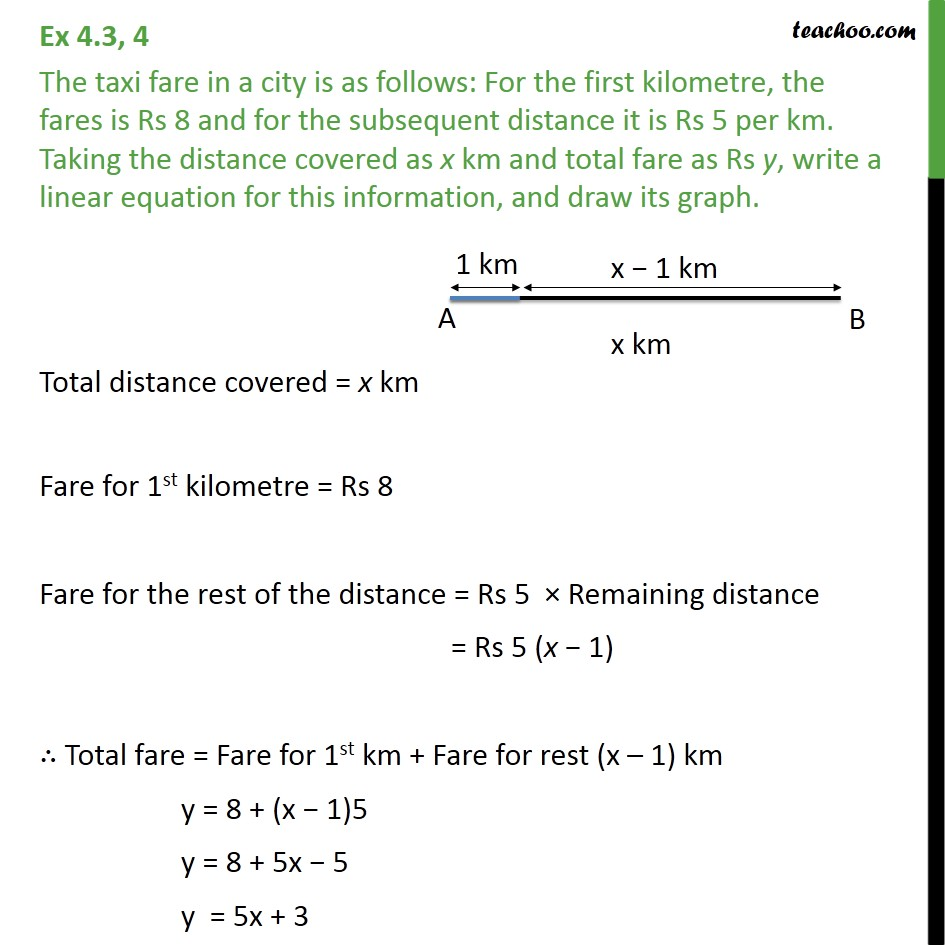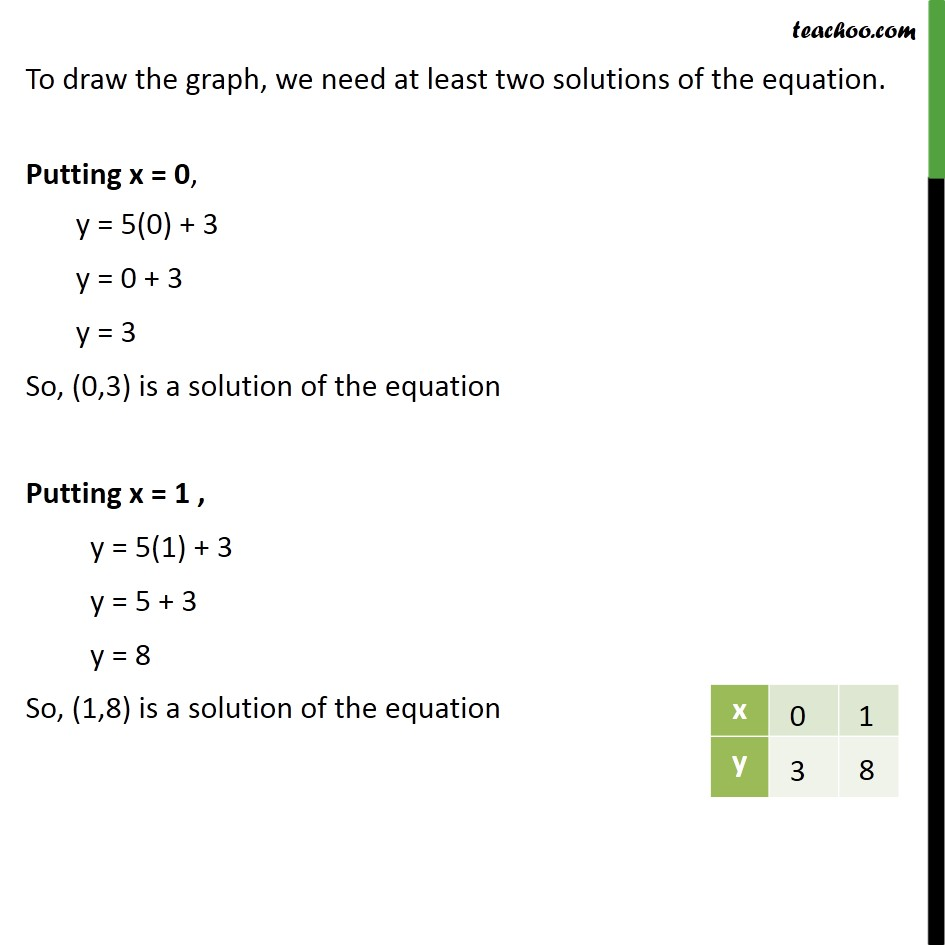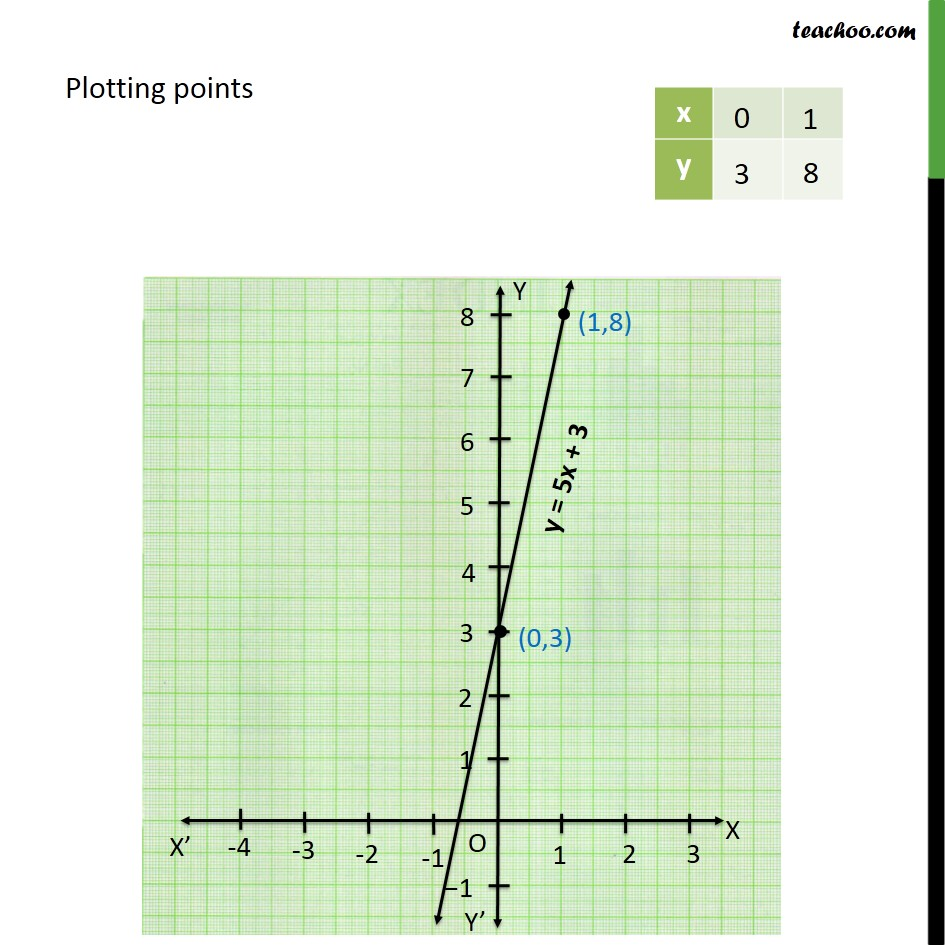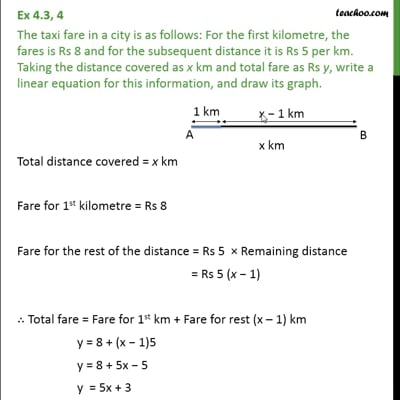Ex 4.3

Chapter 4 Class 9 Linear Equations in Two Variables
Serial order wiseThis video is only available for Teachoo black users

Solve all your doubts with Teachoo Black (new monthly pack available now!)

### Transcript

Ex 4.3, 4 The taxi fare in a city is as follows: For the first kilometre, the fares is Rs 8 and for the subsequent distance it is Rs 5 per km. Taking the distance covered as x km and total fare as Rs y, write a linear equation for this information, and draw its graph. Total distance covered = x km Fare for 1st kilometre = Rs 8 Fare for the rest of the distance = Rs 5 Remaining distance = Rs 5 (x 1) Total fare = Fare for 1st km + Fare for rest (x 1) km y = 8 + (x 1)5 y = 8 + 5x 5 y = 5x + 3 To draw the graph, we need at least two solutions of the equation. Putting x = 0, y = 5(0) + 3 y = 0 + 3 y = 3 So, (0,3) is a solution of the equation Putting x = 1 , y = 5(1) + 3 y = 5 + 3 y = 8 So, (1,8) is a solution of the equation Plotting points